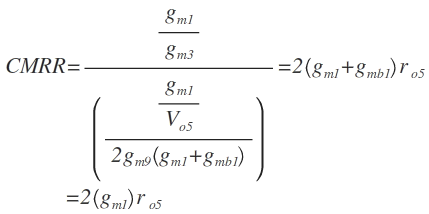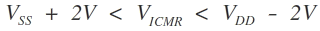Home > Analog CMOS Design > CMOS Differential Amplifier > CMRR

Common mode rejection ratio (CMRR)

The common mode rejection ratio (CMRR) is defined as the ratio of differential mode gain and common mode gain.Input Common mode Range (ICMR):

The input common mode range (ICMR) is defined as the common mode signal range over which the differential voltage gain of the differential amplifier remains constant. The ICMR is given by